# MA2051--C97 --- Solutions for TEST II

1. (a) The roots for the characteristic equation are -1 and 3/2, so two solutions are e-t and e(3/2) t. To verify independence, compute the Wronskian and show that it is not zero.
(b) Use the method of undetermined coefficients to find yp(t) = 1/2 e-3t.
(c) The general solution is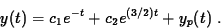The initial data give c1 = -6/5 and c2 = +6/5, so y(t) = (6/5) e (3/2)t - (6/5) e- t + (1/2) e-3t.
(d) For large values of t, the solution approaches the exponential term (6/5)e(3/2)t -- everything else is transient. There is no limiting periodic solution. <\br><\br> 2. (a) The data give you m = 6 and k = (6)(9.8)/(0.02) = 2940, so the model reduces to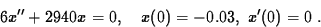(b) The characteristic equation has roots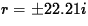(approximately) and so the general solution is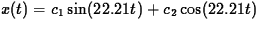. The initial data determine c1 = 0 and c2 = -0.03.
(c) The amplitude is 0.03. The period isand it takes one half of a period to travel from highest position to the lowest position.
(d) The velocity is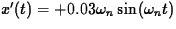and the mass passes through equilibrium at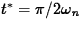. The velocity is therefore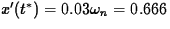(meters per second).

3. (a) The characteristic equation reduces to (r+4)(r-2) = 0 so the roots are r1 = -4 and r2 = +2. The first solution pair is (y1,z1) = (e-4t,3e-4t). The second solution pair is (y2,z2) = (e2t,e2t). (You can test linear independence with the Wronskian.) The general solution is (y,z) = c1(y1,z1) + c2(y2,z2).
(b) The initial data givesand so y= 50e2t, z= 50e2t. (This is a straight line and the solution moves away from the origin as t increases.)
(c) If you choose initial data so that the constants in the general solution becomeand c2 = 0, then you get convergence to the origin; the solution becomes (y,z) = c1(e-4t, 3 e-4t). (You must remove'' that nasty e+2t.) Any initial data of the form y(0) = (1/3) z(0) works. The solution curve in this case follows a straight line to the origin.

4. The critical damping coefficient is p = 4 and the solution in this case is

x(t) = xi e-2t + ( vi + 2xi) t e-2t

Set this equal to zero and solve for exactly one value: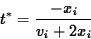If xi > 0, this value of t is positive if and only if vi < -2xi. (You have to push the mass toward the origin to be sure it gets there.)

Arthur Heinricher <heinrich@wpi.edu>
Last modified: Wed Oct 8 08:36:08 EDT 1997